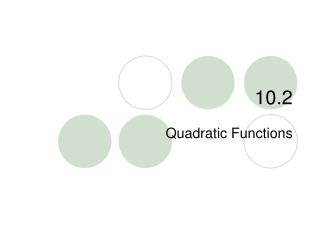DownloadDownload Presentation10.2

# 10.2

Download Presentation## 10.2

- - - - - - - - - - - - - - - - - - - - - - - - - - - E N D - - - - - - - - - - - - - - - - - - - - - - - - - - -
##### Presentation Transcript

2. 10.2 – Quadratic Functions • Goals / “I can…” • I can graph quadratic functions of the form y = ax + bx + c • I can graph quadratic inequalities 2

3. 10.2 – Quadratic Functions 2 • Yesterday we learned about y = ax and y = ax + c. The a changes the ????? and the c moves the parabola ?????. 2

4. 10.2 – Quadratic Functions 2 • Today we look at y = ax + bx + c. The bx moves the parabola horizontally, changing the location of the line of symmetry.

5. 10.2 – Quadratic Functions 2 • In an equation y = ax + bx + c, the line of symmetry can be found by the equation • The x-coordinate of the vertex is

6. Finding the Line of Symmetry 10.2 – Quadratic Functions When a quadratic function is in standard form For example… Find the line of symmetry of y =3x2 – 18x + 7 y = ax2 + bx + c, The equation of the line of symmetry is Using the formula… Thus, the line of symmetry is x = 3 This is best read as … the opposite of b divided by the quantity of 2 times a.

7. Finding the Vertex 10.2 – Quadratic Functions y = –2x2 + 8x –3 We know the line of symmetry always goes through the vertex. STEP 1: Find the line of symmetry STEP 2: Plug the x – value into the original equation to find the y value. Thus, the line of symmetry gives us the x – coordinate of the vertex. y = –2(2)2 + 8(2) –3 y = –2(4)+ 8(2) –3 y = –8+ 16 –3 To find the y – coordinate of the vertex, we need to plug the x – value into the original equation. y = 5 Therefore, the vertex is (2 , 5)

8. USE the equation A Quadratic Function in Standard Form 10.2 – Quadratic Functions The standard form of a quadratic function is given by y = ax2 + bx + c There are 3 steps to graphing a parabola in standard form. MAKE A TABLE using x – values close to the line of symmetry. Plug in the line of symmetry (x – value) to obtain the y – value of the vertex. STEP 1: Find the line of symmetry STEP 2: Find the vertex STEP 3: Find two other points and reflect them across the line of symmetry. Then connect the five points with a smooth curve.

9. 10.2 – Quadratic Functions • Graph the equation y = -3x + 6x + 5 2

10. 10.2 – Quadratic Functions • Graphing Quadratic Inequalities • Graphing a parabola with an inequality is similar to a line. We use solid and dashed lines and we shade above (greater) or below (less) the line.

11. Forms of Quadratic Inequalitiesy<ax2+bx+c y>ax2+bx+cy≤ax2+bx+c y≥ax2+bx+c • Graphs will look like a parabola with a solid or dotted line and a shaded section. • The graph could be shaded inside the parabola or outside.

12. 10.2 – Quadratic Functions 1. Sketch the parabola y=ax2+bx+c (dotted line for < or >, solid line for ≤ or ≥) ** remember to use 5 points for the graph! 2. Choose a test point and see whether it is a solution of the inequality. 3. Shade the appropriate region. (if the point is a solution, shade where the point is, if it’s not a solution, shade the other region)

13. Graph y ≤ x2 + 6x - 4 10.2 – Quadratic Functions Test point * Opens up, solid line * Vertex: (-3,-13) • Test Point: (0,0) • 0≤02+6(0)-4 • 0≤-4 So, shade where the point is NOT!

14. Graph: y > -x2 + 4x - 3 10.2 – Quadratic Functions Test Point * Opens down, dotted line. * Vertex: (2,1) • x y • 0 -3 • 1 0 • 1 • 0 • -3 * Test point (0,0) 0>-02+4(0)-3 0>-3

15. 1) y ≥ x2 and 2)y ≤ -x2 + 2x + 4 10.2 – Quadratic Functions SOLUTION! • Graph both on the same coordinate plane. The place where the shadings overlap is the solution. • Vertex of #1: (0,0) Other points: (-2,4), (-1,1), (1,1), (2,4) • Vertex of #2: (1,5) Other points: (-1,1), (0,4), (2,4), (3,1) * Test point (1,0): doesn’t work in #1, works in #2.

16. 10.2 – Quadratic Functions • Graph: y > x + x+ 1 2